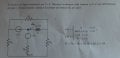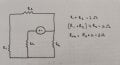# First order circuit

#### Alessia00Ma

Joined Oct 11, 2021
1
Hi, I'm trying to solve a first order circuit but I'm having a lot of trouble. Here's the exercise:The circuit is in steady state for t < 0. I need to find the voltage across the inductor ∀t.
I'm trying to solve this circuit using Norton's (tried Thevenin too) theorem , but I can't find the current jcc that flows through the short-circuited inductor.
I've determined the equivalent resistance at the inductor's terminals, by turning off the independent sourcesThen, I've applied the superposition theorem to find the current jcc.
First, I've turned off the voltage source e(t), so it is short-circuited.
This is where I'm having trouble. How do I find jcc? With the voltage source and the inductor short circuited the resistor R2 and R4 are in parallel, this parallel is in series with the resistor R1 which is in parallel with R3. Is jcc equal j(t)?

I appreciate any help

#### dcbingaman

Joined Jun 30, 2021
855
When you short L you are finding the Norton equivalent circuit constant current. In this case the current must flow R3 that is then in parallel with (R1+R2||R4). So the current source will 'see' R3||(R1+R2||R4). The resistance is what you get looking into the terminals across L. In this case you have already determined that to be 2 ohms. Now you can determine an equivalent voltage source to replace the current source by taking the current and multiplying it by R3||(R1+R2||R4) to get an equivalent voltage source and you have already determined R. I cannot go on without providing you a solution but this should help you get on the right path for an answer.